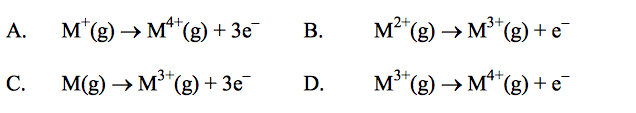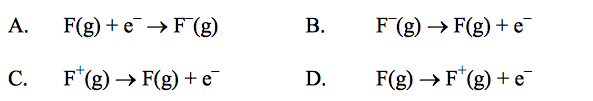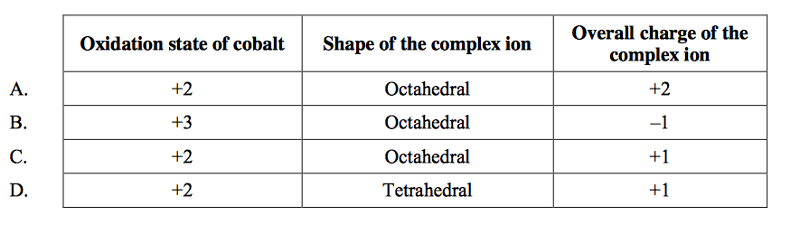# Periodicity Flashcards

Set Details Share
created 11 years ago by hdiwza
6,700 views
updated 11 years ago by hdiwza
Page to share:
Embed this setcancel
COPY
code changes based on your size selection
Size:
X

1

Which pair of elements reacts most readily?
A. Li + Br2
B. Li + Cl2
C. K + Br2
D. K + Cl2

D

2

Which of the following salts form coloured solutions when dissolved in water? I. ScCl3 II. FeCl3 III. NiCl2 IV. ZnCl2
A. I and II only
B. II and III only
C. III and IV only
D. I, II, III and IV

B

3

The compounds Na2O, Al2O3 and SO2 respectively are
A. acidic, amphoteric and basic.
B. amphoteric, basic and acidic.
C. basic, acidic and amphoteric.
D. basic, amphoteric and acidic.

D

4

Which of the following properties of the halogens increase from F to I?
I. Atomic radius II. Melting point III. Electronegativity
A. I only
B. I and II only
C. I and III only
D. I, II, and III

B

5

Which pair would react together most vigorously?
A. Li and Cl2
B. Li and Br2
C. K and Cl2
D. K and Br2

C

6

Which properties of period 3 elements increase from sodium to argon? I. Nuclear charge II. Atomic radius III.Electronegativity
A. I and II only
B. I and III only
C. II and III only
D. I, II, and III

B

7

Which general trends are correct for the oxides of the period 3 elements (Na2O to Cl2O)?
I. Acid character decreases.
II. Electrical conductivity (in the molten state) decreases.
III. Bonding changes from ionic to covalent.
A. I and II only
B. I and III only
C. II and III only
D. I, II, and III

C

8

For which element are the group number and the period number the same?
A. Li
B. Be
C. B
D. Mg

B

9

Which of the physical properties below decrease with increasing atomic number for both the alkali metals and the halogens?
I. Atomic radius II. Ionization energy III. Melting point
A. I only
B. II only
C. III only
D. I and III only

B

10

Which of the reactions below occur as written?
I. Br2 + 2I– → 2Br– + I2 II. Br2 + 2Cl– → 2Br– + Cl2
A. I only
B. II only
C. Both I and II
D. Neither I nor II

A

11

Rubidium is an element in the same group of the periodic table as lithium and sodium. It is likely to be a metal which has a
A. high melting point and reacts slowly with water.
B. high melting point and reacts vigorously with water.
C. low melting point and reacts vigorously with water.
D. low melting point and reacts slowly with water.

C

12

When the following species are arranged in order of increasing radius, what is the correct order?
A. Cl–, Ar, K+
B. K+, Ar , Cl–
C. Cl–, K+, Ar
D. Ar, Cl–, K+

B

13

What increases in equal steps of one from left to right in the periodic table for the elements lithium to neon?
A. the number of occupied electron energy levels
B. the number of neutrons in the most common isotope
C. the number of electrons in the atom
D. the atomic mass

C

14

Which property decreases down group 7 in the periodic table?
B. electronegativity
D. melting point

B

15

Which two elements react most vigorously with each other?
A. chlorine and lithium
B. chlorine and potassium
C. iodine and lithium
D. iodine and potassium

B

16

Which is an essential feature of a ligand?
A. a negative charge
B. and odd number of electrons
C. the presence of two or more atoms
D. the presence of a non-bonding pair of electrons

D

17

Which properties are typical of most non-metals in period 3 (Na to Ar)?
I. They form ions by gaining one or more electrons.
II. They are poor conductors of heat and electricity.
III. They have high melting points.
A. I and II only
B. I and III only
C. II and III only
D. I, II, and III

A

18

A potassium atom has a larger atomic radius than a sodium atom. Which statement about potassium correctly explains this difference?
A. It has a larger nuclear charge.
B. It has a lower electronegativity.
C. It has more energy levels occupied by electrons.
D. It has a lower ionization energy.

C

19Which equation represents the third ionization energy of an element M?

B

20

Which factors lead to an element having a low value of first ionization energy?
II. high number of occupied energy levels
III. high nuclear charge
A. I and II only
B. I and III only
C. II and III only
D. I, II, and III

A

21

Which statement about electronegativity is correct?
A. Electronegativity decreases across a period.
B. Electronegativity increases down a group.
C. Metals generally have lower electronegativity values than non-metals.
D. Noble gases have the highest electronegativity values.

C

22

Which statement is correct for a periodic trend?
A. Ionization energy increases from Li to Cs.
B. Melting point increases from Li to Cs.
C. Ionization energy increases from F to I.
D. Melting point increases from F to I.

D

23

Which compound of an element in period 3 reacts with water to form a solution with a pH greater than 7?
A. SiO2
B. SiCl4
C. NaCl
D. Na2O

D

24

Which electrons are lost by an atom of iron when it forms the Fe3+ ion?
A. One s orbital electron and two d orbital electrons
B. Two s orbital electrons and one d orbital electron
C. Three s orbital electrons
D. Three d orbital electrons

B

25Which equation represents the first ionization energy of fluorine?

D

26

Which properties are typical of d-block elements?
I. complex ion formation II. catalytic behavior III. colorless compounds
A. I and II only
B. I and III only
C. II and III only
D. I, II, and III

A

27

Which statement is correct for the halogen group?
A. Halide ions are all reducing agents, with iodide ions being the weakest.
B. Halogens are all oxidizing agents, with chlorine being the strongest.
C. Chloride ions can be oxidized to chlorine by bromine.
D. Iodide ions can be oxidized to iodine by chlorine.

D

28

Which of the following statements are correct?
I. The melting points decrease from Li -> Cs for the alkali metals.
II. The melting points increase from F -> I for the halogens.
III. The melting points decrease from Na -> Ar for the period 3 elements.
colourless compounds I, II and III
A. I and II only
B. I and III only
C. II and III only
D. I, II, and III

A

29

Which element is a transition metal?
A. Ca
B. Cr
C. Ge
D. Se

B

30

When Na, K, and Mg are arranged in increasing order of atomic radius (smallest first), which order is correct?
A. Na, K, Mg
B. Na, Mg, K
C. K, Mg, Na
D. Mg, Na, K

D

31

Which oxides produce an acidic solution when added to water?
I. SiO2 II. P4O6 III. SO2
A. I and II only
B. I and III only
C. II and III only
D. I, II, and III

C

32

Which combination of ion charge and ion size produces the greatest lattice enthalpy?
A. High charge, large size
B. High charge, small size
C. Low charge, small size
D. Low charge, large size

B

33

Which series is arranged in order of increasing radius?
A. Ca2+ < Cl– < K+
B. K+ < Ca2+ < Cl–
C. Ca2+ < K+ < Cl–
D. Cl– < K+ < Ca2+

C

34

Which salts form coloured solutions when dissolved in water?
I. FeCl3 II. NiCl2 III. ZnCl2
A. I and II only
B. I and III only
C. II and III only
D. I, II, and III

A

35Which combination is correct for the complex ion in [Co(NH3)4(H2O)Cl]Br?

C